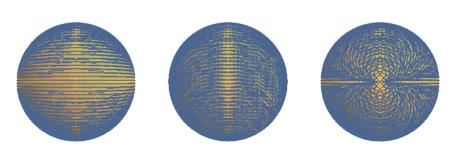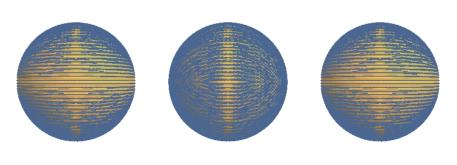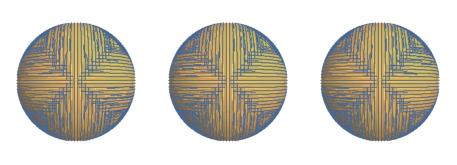This is a quick note about some methods for computing an orthonormal basis from a unit (bi)vector. The math directly follows that in “Implied normals (unit bivectors as rotations)”.

Toy C code can be found: HERE

A re-cap of the needed bits from implied. Start with a standard orthonormal basis set:

$\begin{eqnarray*} \mathbf{x} & = & \left(1,0,0\right) \\ \mathbf{y} & = & \left(0,1,0\right) \\ \mathbf{z} & = & \left(0,0,1\right) \end{eqnarray*}$

Given a unit (bi)vector:

$\mathbf{v} = \left(v_x,~v_y,~v_z\right)$

Then the quaternion $Q$ which rotates $\mathbf{z}$ to $\mathbf{v}$:

$\begin{eqnarray} Q & = & \sqrt{\mathbf{v}\mathbf{z}^*} \nonumber \\ & = & \sqrt{-\mathbf{vz}} \nonumber \\ & = & \sqrt{\mathbf{z} \cdot \mathbf{v} + \mathbf{z} \times \mathbf{v}} \nonumber \\ & = & \sqrt{v_z + \left(-v_y,~v_x,~0 \right)} \nonumber \\ & = & \frac{1+v_z}{\sqrt{2+2v_z}} + \left(\frac{-v_y}{\sqrt{2+2v_z}},~\frac{v_x}{\sqrt{2+2v_z}},~0\right) \label{fx} \end{eqnarray}$

This post gives an informal justification using vectors.

## Single reference direction sticking with up seems reasonable

Directly using $\eqref{fx}$ we can transform (rotate) the standard orthonormal set {$\mathbf{x,y,z}$} into a new set {$\mathbf{x’,y’,v}$}:

$\begin{eqnarray} \mathbf{x}' & = & Q\mathbf{x}Q^* \nonumber \\ & = & \left( v_z+\frac{v_y^2}{v_z+1},~-\frac{v_x v_y}{v_z+1},~-v_x \right) \label{xp} \\ \nonumber \\ \mathbf{y}' & = & Q\mathbf{y}Q^* \nonumber \\ & = & \left( -\frac{v_x v_y}{v_z+1},~1-\frac{v_y^2}{v_z+1},~-v_y \right) \label{yp} \\ \nonumber \\ \mathbf{z}' & = & Q\mathbf{z}Q^* = \mathbf{v} \nonumber \\ \end{eqnarray}$

A simple translation of $\eqref{xp}$ and $\eqref{yp}$ into ‘C’ gives:

Simply inspecting the code or recalling the math shows a problem as $\mathbf{v}$ approaches $-\mathbf{z}$, we have an infinite number of solutions for $Q$ and numerically the math explodes. In cases were this is possible the above could be augmented by adding a branch and setting to xp and yp to two orthogonal vectors in the {$\mathbf{xy}$}-plane or the “correct” values in the limit. Choice of branch point and values depend on use requirements. The next section will provide a branch-free alternate to these cases.

The math basis for this method when using positive $\mathbf{z}$ as a single reference direction is identically to that in Frisvad1. However the math is presented differently and the derivation is carried through differently as well:

$\begin{eqnarray} \mathbf{x_f} & = & \left( 1+\frac{v_x^2}{v_z+1},~-\frac{v_x v_y}{v_z+1},~-v_x \right) \label{frisvad_x} \\ \mathbf{y_f} & = & \left( -\frac{v_x v_y}{v_z+1},~1-\frac{v_y^2}{v_z+1},~-v_y \right) \label{frisvad_y} \end{eqnarray}$

which directly translation into code results at least four instead of two products.

The choice of equations slightly changes the error bounds. Below we have a plot for the full domain where the x-axis is the height of the cap around the reference direction.

If we multiply through either pair of equations by -1 above we can drop a number of computations. This is equivalent to rotating the output by $\pi$ or reworking the original equations starting with a basis set of {$\mathbf{-x,-y,z}$}. Choosing to stick with my form gives:

$\begin{eqnarray} \mathbf{x}' & = & \left( -v_z-\frac{v_y^2}{v_z+1},~\frac{v_x v_y}{v_z+1},~v_x \right) \label{xpr} \\ \nonumber \\ \mathbf{y}' & = & \left( \frac{v_x v_y}{v_z+1},~\frac{v_y^2}{v_z+1}-1,~v_y \right) \label{ypr} \end{eqnarray}$

## Two opposite reference directions up and down, why not?

A second possible solution is to form the rotation with nearest direction of two choices. As an example we can form the rotation that maps $-\mathbf{z}$ to $\mathbf{v}$ and use that when $v_z < 0$:

$\begin{eqnarray} Q_n & = & \sqrt{\mathbf{vz}} \nonumber \\ & = & \frac{1-v_z}{\sqrt{2-2v_z}} + \left(\frac{v_y}{\sqrt{2-2v_z}},~-\frac{v_x}{\sqrt{2-2v_z}},~0\right) \label{nx} \end{eqnarray}$

Rotating $\mathbf{x}$ and $\mathbf{y}$ by $Q_n$:

$\begin{eqnarray} \mathbf{x}' & = & \left( -v_z-\frac{v_y^2}{v_z-1},~\frac{v_x v_y}{v_z-1},~v_x \right) \label{xn} \\ \mathbf{y}' & = & \left( \frac{v_x v_y}{v_z-1},~1+\frac{v_y^2}{v_z-1},~v_y \right) \label{yn} \end{eqnarray}$

The differences between $\eqref{xp} \eqref{yp}$ and $\eqref{xn} \eqref{yn}$ can be expressed by taking the absolute value of $v_z$ and products of $s_z= \text{sgn}\left(v_z\right)$. So we can combine the two expressions as follows:

$\begin{eqnarray} \mathbf{x}' & = & \left( \abs{v_z}+\frac{v_y^2}{\abs{v_z}+1},~-\frac{v_x v_y}{\abs{v_z}+1},~-s_z v_x \right) \label{xpn} \\ \mathbf{y}' & = & \left(-\frac{v_x v_y}{\abs{v_z}+1},~1-\frac{v_y^2}{\abs{v_z}+1},~-s_z v_y \right) \label{ypn} \end{eqnarray}$

This method has a potential issue since $Q_n$ maps $-\mathbf{z}$ to $\mathbf{v}$. This means the source basis set is {$\mathbf{x,y,-z}$} when $v_z < 0$ which is left-handed2. This is not an issue if we simply need two mutually orthogonal normals in the complement plane, however for cases where it is an issue we can simply negate one of the computed normals in the $v_z < 0$ case which is equivalent to multiplying through by $s_z$. For both we then have:

$\begin{eqnarray} s_z \mathbf{x}' & = & \left( v_z+\frac{s_z v_y^2}{\abs{v_z}+1},~-\frac{s_z v_x v_y}{\abs{v_z}+1},~-v_x \right) \label{xpnn} \\ s_z \mathbf{y}' & = & \left(-\frac{s_z v_x v_y}{\abs{v_z}+1},~s_z-\frac{s_z v_y^2}{\abs{v_z}+1},~-v_y \right) \label{ypnn} \end{eqnarray}$

Choosing to use $s_z \mathbf{x}’$ gives us:

## Pixar’s method and inspiration thereof

A recently published paper from Pixar3 presents a new method directly derived from that of Frisvad $\eqref{frisvad_x} \eqref{frisvad_y}$ which is both efficient and high quality. Translating their branch-free version into equations we have:

$\begin{eqnarray} \mathbf{x_p} & = & \left( 1-\frac{s_z v_x^2}{v_z+s_z},~-\frac{s_z v_x v_y}{v_z+s_z},~-s_z v_x \right) \label{pixar_x} \\ \mathbf{y_p} & = & \left( -\frac{v_x v_y}{v_z+s_z},~s_z-\frac{v_y^2}{v_z+s_z},~-v_y \right) \label{pixar_y} \end{eqnarray}$

Over reals these are equivalent to $\eqref{xpn}$ and $\eqref{ypnn}$ (so it returns the opposite results of the 2a method above). Their strategy for handling the negative half sphere is obviously superior to the one I used above. Where I was computing both abs and sign of z, they only use the latter. The transform is simple: multiply top and bottom through by $s_z$:

$\frac{a}{\abs{v_z}+1} = \frac{s_z a}{v_z+s_z}$

After the palmface I wanted to see what could be done with the new puzzle piece.

Starting with Pixar’s method we can negate both the returned vectors and on X64 hardware this lowers the number of issues from 36 to 324. Additionally both GCC and clang fail to remove the redundant sz*x term which bring us to 30:

For X86 we’ve already beaten method 2a in terms of issues, which requires 32.

If we can allow left-handed results on one of the half-spheres then we can multiply the $\mathbf{x}’$ result of the previous through by $s_z$ which lowers the number of X64 issues to 28 (same as lower quality method 2 above):

So far we haven’t changed the error. Since we are not allowing the compiler to treat FP as reals there are some redundant computations given the order of operations. Choosing to pull out y*a drops the number of issues to 29/26 respectively and my RMS measures5 show a minor negative impact6 of ~0.003%. (Both compilers figure out the sz*x terms here)

That exhasts the things that seem profitable and jump out at me to try working with x component reduction used in $\eqref{frisvad_x}$.

Returning the my previous choice of x-component expansion: take $\eqref{xpnn} \eqref{ypnn}$, change to Pixar’s strategy and negating both give a left-handed system on one half sphere.

and multiply $\mathbf{y}’$ by $s_z$ for right handed version:

Note I’m only using X86 issues as a quick-n-dirty measure of goodness (easy to copy-n-past into godbolt).

A summary of results:

method x86-64 RMS rel-RMS notes
basis_2 28 2.5868×10-8 -7.028%
basis_2a 32 2.5868×10-8 -7.028%
pixar 36 2.4170×10-8 - as per paper
pixar_r1 30 2.4170×10-8 -
pixar_r2 29 2.4215×10-8 -0.003%
pixar_l1 28 2.4170×10-8 -
pixar_l2 26 2.4215×10-8 -0.003%
basis_r1 27 2.5868×10-8 -7.028%
basis_l2 24 2.5868×10-8 -7.028%

## Other methods

Thanks to Stephen Hill for providing references to some existing methods and (better yet) for writting up a summary in a blog post7 and a link to a second pair of methods by Sam Hocevar8.

These methods are all roughly: Given $\mathbf{v}$ inspect the components and choose vector $\bar{\mathbf{w}}$ based on them. Cross product creates an orthgonal vector. This effectively partitions the sphere into “combing” groups.

The method above can be extended at increased computational complexity to shape how the normals are combed. As an example we could add a twist in {$\mathbf{x,y}$} like that found in a Wiechel projection or any of a number of craziness.

## Viz

These figures roughly show the behavior of one output normal. I’m not finding a quick-and-dirty viz that I really like. Down positive z, positive x and negative z.

Method 1: Minimal angle orientation change on sphere.Method 2: Minimal angle orientation change on two half spheres.Hughes Moller:## References and Footnotes

1. “Building an orthonormal basis from a 3d unit vector without normalization”, Jeppe Frisvad, Journal of Graphics Tools 16:33, (2012) PDF/code page

2. If you don’t like “handedness” then $\mathbf{x}’ \times \mathbf{y}’ = -\mathbf{v}$ instead of $\mathbf{v}$.

3. “Building an Orthonormal Basis, Revisited”, Tom Duff, James Burgess, Per Christensen, Christophe Hery, Andrew Kensler, Max Liani, and Ryusuke Villemin, Journal of Computer Graphics Techniques Vol. 6, No. 1, 2017 JCGT

4. Instruction issue numbers are from x86-64 gcc 6.3 with -O3. x86-64 clang 4.0.0 -O3 numbers appear to be the same.

5. Take with a grain-of-salt. My RMS measures are not in agreement with those reported by Pixar.

6. Computed the standard way: $1-\frac{e}{e_0}$

7. “Perpendicular Possibilities”, Stephen Hill, 2011 blog post

8. “On picking an orthogonal vector (and combing coconuts)”, Sam Hocevar, 2013 blog post